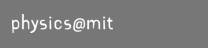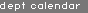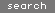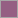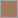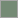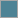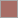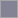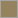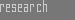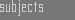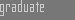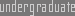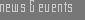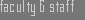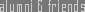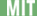> Home > General Info > Experiments > Problem Sets > Quizzes & Exams > FAQs > Staff > Feedback > Grades > Lecture Notes##8.02X :: Electricity & MagnetismLatest News5/6/05 [2:10PM]

Lecture slides from today are here.

5/4/05 [4:00PM]

Solutions to quiz 4 are here.

5/3/05 [9:45PM]

Because of the exam, tutoring will be held today instead of tomorrow. The hours will be 5-7 pm.

5/2/05 [5:35PM]

Solutions to practice quiz 4b are here.

5/2/05 [12:00PM]

Solutions to PS 11, PS 9 and experiment AMP are here. Today's Quiz 4 review is here.

5/1/05 [11:59PM]

Solutions to practice quiz 4a are here.

4/30/05 [2:20PM]

Second practice quiz 4 has been posted here.

4/29/05 [2:50PM]

Lecture slides from today are here.

4/27/05 [4:20PM]

Pset 12 (experiment MW section) has been posted here.
4/27/05 [10:40AM]
Practice quiz #4 is here.

4/24/05 [8:00PM]

Pset 11 has been posted here.

4/20/05 [7:50PM]

Lecture slides from today are here.

4/20/05 [2:50PM]

Solutions to quiz #3 are here.

4/17/05 [4:00PM]

Pset 10 has been posted here.

4/14/05 [3:20PM]

Slides from yesterdays lecture can be found here.

4/10/05 [11:30PM]

Solutions for exp MF have been posted here. Rules for formula sheets are the same as for previous quizzes.
4/10/05 [2:30PM]

Solutions for practice quiz 3b have been posted here. I fixed the solutions for 3a, problem 4a. Review session will take place in 24-402 from 3PM to 8PM today.
4/10/05 [11:40AM]

Practice quiz 3b has been posted here. Solutions for pset 8 have been posted here.

4/10/05 [9:25AM]

Solutions to Practice Quiz #3a:
• 1a) Free electrons that enter the high field in the spark gap are accelerated. If their mean free path is big enough and the field is high enough, their kinetic energy may become big enough to ionize air molecules they collide with. In the collision process, further electrons will be released, which leads to an avalanche and electric breakdown (a discharge) across the gap.
• 1b) Electric Field E = V_gap/d => E = 10MV/m.
• 1c) Potential difference V = E * d => V = 10^10 V/m.

• 2a) The electric field lines run straight from the left (+) to the right (-) plate, with uniform spacing, with no electric field outside the capacitor.
• 2b) Using the right hand rule, one finds that the particles will move on a downward semi-circle upon entering the magnetic field.
• 2c) While traversing the electric field, the kinetic energy of the particle will increase linearly from L=0 to L=1mm. For L>1mm (inside the magnetic field), the particle speed will not change (constant magnetic field does no work) and U_kin will be constant.
• 2d) A particle with 4 times the charge will have a 4 times higher kinetic energy when exiting the capacitor and entering the magnetic field. As U_kin = p^2/m, the momentum will be two times higher. As R = p/(q*B), the radius will be two times smaller.

• 3a) The constant current implies a constant induced EMF and therefore constant dPhi/dt, where Phi is the magnetic flux. As the field is constant, the rod must be moving at a constant velocity to cause this dPhi/dt. A counter-clockwise current means that the magnetic field created by the induced current is in the same direction as the external field. Therefore the flux must be decreasing, which means that the rod is moving to the left.
• 3b) The changing magnetic field would cause a changing magnetic flux and therefore an induced current. As the magnitude of the magnetic flux due to the external field is decreasing, the magnetic field created by the rod would counteract that change and point in the same direction as the external field. Therefore the current would be counter-clockwise. The right-hand rule shows that there would be a force to the right on the rod, accelerating the rod to the right.

• 4a) The corkscrew rule shows that at the right most point, for d << R, the field will point ot the right, away from the the loop .
• 4b) Pick a circular loop for Amperes Law around loop 1. As B(r) constant and B_vec || to r_vec, one can move B(r) outside of the integral and gets B(r)*2pi*r = mu_0 * I and therefore B(r) = mu_0/2*pi * I/r. Note that r is the distance from loop 1, not it's radius R.
• 4c) Force on a current element in loop 2: dF = I dl * B = mu_0/2*pi I^2/d dl. Total force on loop 2: F = mu_0 I^2/d * R, downwards (repulsion, as the currents run in opposite direction).

4/10/05 [12:10AM]

Slides from todays quiz 3 review have been posted here.

4/8/05 [3:30PM]

Quiz 3 practice: Please check the Mastering Physics webpage for practice problems relevant to quiz #3!

4/7/05 [3:00PM]

4/6/05 [8:20PM]

Pset 9 with AMP questions can be found here.

4/5/05 [1:45PM]

Practice quiz #3a can be found here
Reading suggestions for the material from 4/4 and 4/6:
• Mon, 4/4: 29-1 to 29-3
• Wed, 4/6: 29-5 to 29-6
Slides from yesterdays lecture can be found here.

4/2/05 [3:00AM]

Pset 8 with MF questions can be found here.
> Previous Announcements

[top of page]